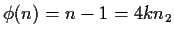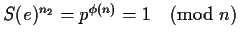Next: Simple algebraic relations Up: Modular mappings Previous: Trigonometrics and complex arguments

## A better method for exponentials and trigonometrics

The previous reasoning, in particular the desire of computing the signatures of exponentials with the largest possible range, prevents us from representing i in the base and in the exponent. This can be overcome if we choose S(e) to be a non-primitive root.

Lets assume that n=4kn2+1 is a prime, and thatis also a prime. This condition is possible to satisfy, and these numbers are similar in concept to safe primes. With them we can represent Sn(i) and also Sn2(i). Now we will choose S(e)=p4k, where p is a primitive root (mod n). By construction,, sofor any choice of S(e). This means that the exponent arithmetic can be done (mod n2), which implies that we can compute the signatures of the exponents in a field. This has many advantages, among others the ability to represent iand being free of any bad divisors. The counterpart is that the signatures of ex will have a smaller set of possible values, n2 instead of n-1, or a factor of 4k. Assuming that k is not too large (it could just be 1), we need to choose n a factor of 4k larger than what we would have done. This is a small price to pay for being able to compute exponent signatures in a field.

As before, we will select S(e) so that it does not satisfy any simple algebraic relationship. In this case we want to select it also such that S(e)i does not satisfy any simple relation. For example, if we choose n=4000133 and n2=1000033, which satisfy the above constraints, p=1351050 is a primitive root (mod n) which gives S(e)=266466. Neither S(e) nor S(e)i satisfy any simple algebraic relation.Next: Simple algebraic relations Up: Modular mappings Previous: Trigonometrics and complex arguments
Gaston Gonnet
1999-07-04